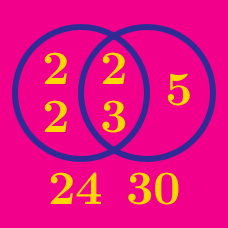Number Theory

# Greatest Common Divisor / Lowest Common Multiple: Level 2 Challenges

$\large n! + 1 \qquad \qquad (n+1)! + 1$

If $n$ is a positive integer, find the greatest common divisor of the two numbers above.

Notation: $!$ denotes the factorial notation. For example, $8! = 1\times2\times3\times\cdots\times8$.

Find the sum of all natural numbers $n$ such that

$\text{lcm}(1,2,3,\ldots, n) = n!$

Notations:

• $\text{lcm}(\cdot)$ denotes the lowest common multiple function.
• $!$ is the factorial notation. For example, $8! = 1\times2\times3\times\cdots\times8$.

If $x$ and $y$ are integers, then which of the following expressions could be equal to 2018?

\begin{aligned} (\underbrace{7+7+7+\cdots+7}_{46 \text{ times}}) - (\underbrace{29+29+29+\cdots+29}_{11 \text{ times}}) &= 3 \\\\ (\underbrace{7+7+7+\cdots+7}_{21 \text{ times}}) - (\underbrace{29+29+29+\cdots+29}_{5 \text{ times}}) &= 2 \end{aligned}

Is it also possible to find positive integers $m$ and $n$ such that $(\underbrace{7+7+7+\cdots+7}_{m \text{ times}}) - (\underbrace{29+29+29+\cdots+29}_{n \text{ times}}) = 1 ?$

Positive integers from 1 to 3141 (inclusive) are written on a blackboard. Two numbers from the board are chosen, erased, and their greatest common divisor is written.

This is repeated until only one number remains on the blackboard. What is the maximum possible value of this number?

×